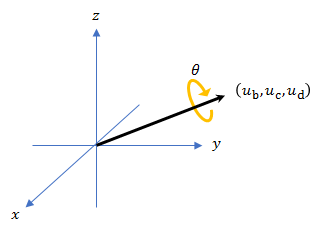# dist

## Syntax

``distance = dist(quatA,quatB)``

## Description

example

````distance = dist(quatA,quatB)` returns the angular distance in radians between two quaternions, `quatA` and `quatB`.```

## Examples

collapse all

Calculate the quaternion distance between a single quaternion and each element of a vector of quaternions. Define the quaternions using Euler angles.

`q = quaternion([0,0,0],'eulerd','zyx','frame')`
```q = quaternion 1 + 0i + 0j + 0k ```
`qArray = quaternion([0,45,0;0,90,0;0,180,0;0,-90,0;0,-45,0],'eulerd','zyx','frame')`
```qArray=5×1 quaternion array 0.92388 + 0i + 0.38268j + 0k 0.70711 + 0i + 0.70711j + 0k 6.1232e-17 + 0i + 1j + 0k 0.70711 + 0i - 0.70711j + 0k 0.92388 + 0i - 0.38268j + 0k ```
`quaternionDistance = rad2deg(dist(q,qArray))`
```quaternionDistance = 5×1 45.0000 90.0000 180.0000 90.0000 45.0000 ```

If both arguments to `dist` are vectors, the quaternion distance is calculated between corresponding elements. Calculate the quaternion distance between two quaternion vectors.

```angles1 = [30,0,15; ... 30,5,15; ... 30,10,15; ... 30,15,15]; angles2 = [30,6,15; ... 31,11,15; ... 30,16,14; ... 30.5,21,15.5]; qVector1 = quaternion(angles1,'eulerd','zyx','frame'); qVector2 = quaternion(angles2,'eulerd','zyx','frame'); rad2deg(dist(qVector1,qVector2))```
```ans = 4×1 6.0000 6.0827 6.0827 6.0287 ```

Note that a quaternion represents the same rotation as its negative. Calculate a quaternion and its negative.

`qPositive = quaternion([30,45,-60],'eulerd','zyx','frame')`
```qPositive = quaternion 0.72332 - 0.53198i + 0.20056j + 0.3919k ```
`qNegative = -qPositive`
```qNegative = quaternion -0.72332 + 0.53198i - 0.20056j - 0.3919k ```

Find the distance between the quaternion and its negative.

`dist(qPositive,qNegative)`
```ans = 0 ```

The components of a quaternion may look different from the components of its negative, but both expressions represent the same rotation.

## Input Arguments

collapse all

Quaternions to calculate distance between, specified as comma-separated quaternions or arrays of quaternions. `quatA` and `quatB` must have compatible sizes:

• `size(quatA) == size(quatB)`, or

• `numel(quatA) == 1`, or

• `numel(quatB) == 1`, or

• if `[Adim1,…,AdimN] = size(quatA)` and `[Bdim1,…,BdimN] = size(quatB)`, then for `i = 1:N`, either `Adimi==Bdimi` or `Adim==1` or `Bdim==1`.

If one of the quaternion arguments contains only one quaternion, then this function returns the distances between that quaternion and every quaternion in the other argument.

Data Types: `quaternion`

## Output Arguments

collapse all

Angular distance in radians, returned as an array. The dimensions are the maximum of the union of `size(quatA)` and `size(quatB)`.

Data Types: `single` | `double`

## Algorithms

The `dist` function returns the angular distance between two quaternions.

A quaternion may be defined by an axis (ub,uc,ud) and angle of rotation θq: $q=\mathrm{cos}\left({\theta }_{q}}{2}\right)+\mathrm{sin}\left({\theta }_{q}}{2}\right)\left({u}_{b}\text{i}+{u}_{c}\text{j}+{u}_{d}\text{k}\right)$.Given a quaternion in the form, $q=a+b\text{i}+c\text{j}+d\text{k}$, where a is the real part, you can solve for the angle of q as ${\theta }_{q}=2{\mathrm{cos}}^{-1}\left(a\right)$.

Consider two quaternions, p and q, and the product $z=p*\text{conjugate}\left(q\right)$. As p approaches q, the angle of z goes to 0, and z approaches the unit quaternion.

The angular distance between two quaternions can be expressed as ${\theta }_{\text{z}}=2{\mathrm{cos}}^{-1}\left(\text{real}\left(z\right)\right)$.

Using the `quaternion` data type syntax, the angular distance is calculated as:

`angularDistance = 2*acos(abs(parts(p*conj(q))));`

## Extended Capabilities

### Objects

Introduced in R2020a